Reach Us+44 1704 335730
Hybrid Differential Evolution and Harmony Search for Optimal Power Flow | OMICS International
Global Journal of Technology and Optimization
All submissions of the EM system will be redirected to Online Manuscript Submission System. Authors are requested to submit articles directly to Online Manuscript Submission System of respective journal.

# Hybrid Differential Evolution and Harmony Search for Optimal Power Flow

Dinh Luong Le1, Dac Loc Ho1 and Ngoc Dieu Vo2*

1Faculty of Mechanical-Electrical–Electronic, Ho Chi Minh City University of Technology (Hutech), Vietnam

2Department of Power Systems, Ho Chi Minh City University of Technology, Ho Chi Minh City, Vietnam

Corresponding Author:
Ngoc Dieu Vo
Department of Power Systems
Ho Chi Minh City University of Technology
Ho Chi Minh City, Vietnam
E-mail: [email protected]

Received date: January 20, 2015; Accepted date: January 31, 2015; Published date: February 07, 2015

Citation: Le DL, Ho DL, Vo ND (2015) Hybrid Differential Evolution and Harmony Search for Optimal Power Flow. Global J Technol Optim 6:175. doi: 10.4172/2229-8711.1000175

Copyright: © 2015 Le DL, et al. This is an open-access article distributed undermthe terms of the Creative Commons Attribution License, which permits unrestricted use, distribution, and reproduction in any medium, provided the original author and source are credited.

Visit for more related articles at Global Journal of Technology and Optimization

#### Abstract

In this paper, we presents a novel approach for solving optimal power flow (OPF) problems using a hybrid differential evolution and harmony search (DEHS). The DEHS method is an improved differential evolution method based on the harmony search scheme. Harmony Search has strong and easy to combine with other methods in optimization and the Differential Evolution algorithm has a very great ability to search solutions with a fast speed to converge, contrary to the most meta-heuristic algorithms. The DEHS method has the flexible adjustment of the parameters to get a better optimal solution. Moreover, an effective constraint handling framework in the method is employed for properly handling equality and inequality constraints of the problem. The proposed DEHS has been tested on three systems including IEEE-30 bus system with quadratic fuel cost function, IEEE-30 bus system with valve point effects fuel cost function and IEEE-57 bus system with quadratic fuel cost function. The obtained results from DEHS algorithm have been compared to those from other methods in the literature. The result comparison has indicated that the proposed DEHS method is more effective than many other methods for obtaining the optimal solution for the test systems. Therefore, the proposed DEHS is a very favorable method for solving the optimal power flow problems.

#### Keywords

Differential evolution; Harmony search; Optimal power flow

#### Introduction

Optimal power flow (OPF) problem is the important fundamental issues in power system operation. In essence, it is the optimization problem and its main objective is to reduce the total generation cost of units while satisfying unit and system constraints. Although the OPF problem developed long time ago but so far it has been extensively studied due to its importance in power system operation. There have been many methods developed to solve OPF problem from classical methods such as Newton’s method, gradient search, linear programming (LP), nonlinear programming, quadratic programming (QP), etc to methods based on artificial intelligence and evolutionary based methods such as ant colony optimization (ACO), genetic algorithm (GA), improved evolutionary programming (IEP), tabu search (TS), simulated annealing (SA), etc. These methods have been effectively for solving the problem.

#### Optimal Power Flow Problem

The OPF problem can be described as an optimization (minimization) process with nonlinear objective function and nonlinear constraints . The general OPF problem can be expressed as

Minimize F(x) (1)

subject to g(x) = 0 (2)

h(x) ≤ 0(3)

where F(x) the objective function, g(x) represents the equality constraints, h(x) represents the inequality constraints and x is the vector of the control variables, that is those which can be varied by a control center operator (generated active and reactive powers, generation bus voltage magnitudes, transformers taps, etc.).

The essence of the optimal power flow problem resides in reducing the objective function and simultaneously satisfying the load flow equations (equality constraints) without violating the inequality constraints.

The fuel cost function is given by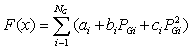(4)

where, NG is the number of generation including the slack bus. PG is the generated active power at bus i. ai, bi and ci are the unit costs curve for ith generator.

The smooth quadratic fuel cost function without valve point loadings of the generating units are given by (4), where the valvepoint effects are ignored. The generating units with multi-valve steam turbines exhibit a greater variation in the fuel-cost functions. Since the valve point results in the ripples, a cost function contains higher order nonlinearity. Therefore, the equation (4) should be replaced by (5) for considering the valve-point effects .

The sinusoidal functions are thus added to the quadratic cost functions as follows .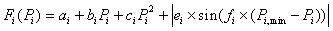(5)

where ai, bi, ci are the fuel cost coefficients of the ith unit and ei and fiare the fuel cost coefficients of the ith unit with valve point effects.

While minimizing the cost function, it is necessary to make sure that the generation still supplies the load demands plus losses in transmission lines. Usually the power flow equations are used as equality constraints .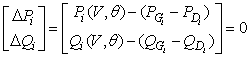(6)

where active and reactive power injection at bus i are defined in the following equation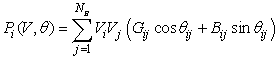(7)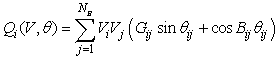(8)

The inequality constraints of the OPF reflect the limits on physical devices in power systems as well as the limits created to ensure system security. The most usual types of inequality constraints are upper bus voltage limits at generations and load buses, lower bus voltage limits at load buses, reactive power limits at generation buses, maximum active power limits corresponding to lower limits at some generators, maximum line loading limits and limits on tap setting. The inequality constraints on the problem variables considered include

Generation constraint: Generator voltages, real power outputs and reactive power outputs are restricted by their upper and lower bounds as follows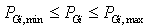for i = 1, 2, . . . . . , NG (9)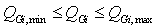for i = 1, 2, . . . . . , NG (10)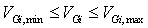for i = 1, 2, . . . . . , NG(11)

Shunt VAR constraint: Shunt VAR compensations are restricted by their upper and lower bounds as follows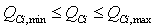for i = 1, 2, . . . . . , NC(12)

where NC is the number of shunt compensator.

Transformer constraint: Transformer tap settings are restricted by their upper and lower bounds as follows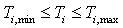for i = 1, 2, . . . . . , NT(13)

where NT is the number of transformer tap.

Security constraint: Voltages at load bus are restricted by their upper and lower bounds as follows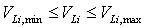for i = 1, 2, . . . . . , NL(14)

where NL is the number of load bus.

#### Hybrid Differential Evolution and Harmony Search

Differential evolution

In 1995, Price and Storn proposed a new evolutionary algorithm for global optimization and named it as differential evolution (DE) . This method has few parameters for tuning make the algorithm quite popular in the literature. DE obtains solutions to optimization problems using three basic operations including mutation, crossover, and selection. The steps of DE including the operations are described as follows:

Step 1: Mutation

Choose the target vector xi,g (= x0,g) and basic vector xr0,g (= x2,g).

Randomly select two vector components xr1,g (= x3,g) and xr2,g (= xNp-2,g).

Calculate the value for a mutant vector: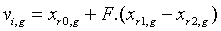(15)

Step 2: Crossover

Select new vector from the target vector and mutant vector according to the following rules: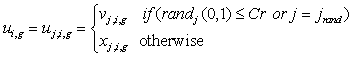(16)

Step 3: Selection

Calculate the value of the objective function with the new vector is created, compare this value to the objective function value of the first selected target vector to decide whether to select a new vector or not.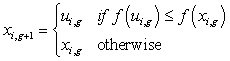(17)

#### Harmony search

Harmony search (HS) algorithm was recently developed in an analogy of music improvisation process where music players improvise the pitches of their instruments to obtain better harmony . First of all, HS initializes harmony memory (HM) which stores the feasible vectors that are all in the feasible space. The harmony memory size (HMS) determines the number of vectors to be stored. Then, through the harmony memory considering rate (HMCR), HS choose any one value from the HM, utilize the pitch adjusting rate (PAR), choose an adjacent value of one value from the HM, and choose totally random value from the possible value range . The steps in the procedure of HS are as follows:

Step 1: Initialize the algorithm parameters and optimization operators such as HMS, HMCR, and PAR.

Step 2: Harmony memory (HM) initialization.

Step 3: Improvise a new harmony from HM. A new harmony vector is generated from HM based on based on three rules: memory considerations, pitch adjustments, and randomization. The HMCR, which varies between 0 and 1, is the probability of choosing one value from the historic values stored in the HM, and (1-HMCR) is the probability of randomly choosing one feasible value not limited to those stored in the HM.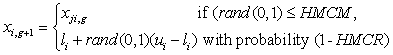(18)

The pitch adjusting process is performed only after a value is chosen from the HM. The value PAR sets the rate of doing pitch adjusting and the value (1-PAR) sets the rate of doing nothing.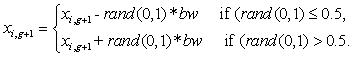(19)

where bw is an arbitrary distance bandwidth (a scalar number) and rand() is a uniformly distributed random number between 0 and 1.

Step 4: Update HM. If the new harmony vector is better than the worst harmony in HM, judged in terms of the objective function value, the new harmony is included in HM and the existing worst harmony is excluded from HM.

Step 5: Repeat Steps 3 and 4 until the terminating criterion is satisfied.

Hybrid differential evolution and harmony search

The overall procedure of the proposed algorithm can be summarized as in Figure 3.

#### Numerical Results

The proposed DEHS algorithm has been applied to OPF problems in three different power systems including IEEE-30 bus system with quadratic fuel cost function, IEEE-30 bus system with valve point effects fuel cost function and IEEE-57 bus system with quadratic fuel cost function. For each system, the proposed DEHS method is run 100 independent trials and the obtained optimal results are compared to those from other methods.

Case 1: The IEEE 30-bus system with quadratic fuel cost function.

In this example, the standard IEEE 30-bus system has been used to test the OPF problems. This system has six generators located at In this example, the standard IEEE 30-bus system has been used to test the OPF problems. This system has six generators located at Figure 2: The procedure of DE . Figure 3: Flow-chart of the DEHS algorithm . Page 4 of 6 Citation: Le DL, Ho DL, Vo ND (2015) Hybrid Differential Evolution and Harmony Search for Optimal Power Flow. Global J Technol Optim 6: 175. doi: 10.4172/2229-8711.1000175 Global J Technol Optim Volume 6 • Issue 2 • 1000175 ISSN: 2229-8711 GJTO, an open access journal buses 1, 2, 5, 8, 11, 13 and four transformers with off-nominal tap ratio in lines 6-9, 6-10, 4-12 and 28-27. Table 1 shows the optimal dispatches of the generators. Also note that all outputs of generator are within its permissible limits. The proposed method is run 100 independent trials and the obtained optimal results are compared to those from other methods such as Genetic algorithm (GA) , tabu search/simulated annealing (TS/SA) , Evolutionary Programming (EP)  and Differential Evolution (DE). It is observed that DEHS algorithm gives better total cost than other methods in a clearly manner. The computation time of the DEHS method is also faster than other methods. These results have shown that the proposed method is feasible and indeed capable of acquiring better solution. Figure 4 shows the convergence characteristic of DE and DEHS. The security constraints are also checked for voltage magnitudes in Figure 5. The voltage magnitudes are between their minimum and maximum values. No load bus was at the lower limit of the voltage magnitudes.

Figure 4: Convergence characteristic of DE and DEHS in IEEE 30- bus system with quadratic fuel cost function.

GA  TS/SA  EP  DE DEHS
Pg1 (MW) 183.1800 192.6244 173.848 179.3918 177.0158
Pg2 (MW) 43.9700 48.4195 49.998 48.1507 48.3280
Pg5 (MW) 18.4400 19.5575 21.386 21.8934 21.8674
Pg8 (MW) 25.6200 11.6716 22.630 20.9393 21.6914
Pg11 (MW) 10.4300 10.0000 12.928 10.0000 11.2231
Pg13 MW) 12.000 12.0000 12.000 12.0257 12.0000
Total loss (MW) - 10.8730 - 9.0008 8.7256
Total cost (\$/h) 805.0132 804.7837 802.62 800.0538 799.5762
Times(s) - - 51.4 14.587 9.657

Table 1: Result comparison of the ieee 30-bus system with quadratic fuel cost
function.

Case 2: The IEEE 30 bus system with valve point effects fuel cost function

In this case, the generating units of buses 1 and 2 are considered to have the valve-point effects on their fuel cost characteristics. The fuel cost coefficients of these generators are taken from . The fuel cost coefficients of the remaining generators have the same values as of the test Case 1. The total cost obtained by the proposed DEHS is less than that from Improved Evolutionary Programming (IEP) , Self- Adaptive Differential Evolution with Augmented Lagrange Multiplier method (SADE_ALM) , Self Organizing Hierarchical Particle Swarm Optimizer with Time Varying Acceleration Coefficients (SOHPSO TVAC)  and Differential Evolution (DE).

The result comparison in Table 2 has indicated that the DEHS algorithm gives better results than other methods with the percentage as follow IEP 2.49%, SADE-ALM 1.47%, SOH – PSO TVAC 0.05% and DE 0.04%. Therefore, the proposed DEHS is very effective for solving the OPF problem with valve point loading effects. Table 2 also show DEHS required the least solution time (Figure 6).

IEP


SOH-PSO
TVAC

DE DEHS
Pg1(MW) 149.7331 193.2903 198.7207 198.2400 199.3440
Pg2(MW) 52.0571 52.5735 50.0361 51.2774 50.6223
Pg5(MW) 23.2008 17.5458 15 15.0000 15.0000
Pg8(MW) 33.4150 10 10 10.0000 10.0000
Pg11(MW) 16.5523 10 10 10.0000 10.0000
Pg13(MW) 16.0875 12 12 12.0193 12.0000
Ploss(MW) 7.6458 12.0096 12.3568 13.5663 13.1367
Total cost (\$/h) 953.573 944.031 930.8860 930.7427 930.3583
Times (s) 93.583m 16.160m 28.24s 20.566 s 13.983s

Table 2: Result comparison of the ieee 30-bus system with valve point effects fuel cost function.

Figure 6: Convergence characteristic of DE and DEHS in IEEE 30- bus system with valve point effects fuel cost function.

Case 3: The IEEE 57 bus system with quadratic fuel cost function (Figure 7).

Case 3: The IEEE 57 bus system with quadratic fuel cost function (Figure 7). To evaluate the effectiveness and efficiency of the proposed DEHS approach in solving larger power system, a standard IEEE 57-bus test system is considered. The IEEE 57-bus system consists of 7 generation buses, 50 load buses, and 80 branches. The generators are located at buses 1, 2, 3, 6, 8, 9, and 12 and 15 transformers are located at branches 19, 20, 31, 37, 41, 46, 54, 58, 59, 65, 66, 71, 73, 76, and 80. The system has also 3 switchable capacitor banks installed at buses 18, 25, and 53. For dealing with this system, there 31 control variables to be handled including real power output of 6 generators except the generator at the slack bus, voltage at 7 generation buses, tap changer of 15 transformers, and reactive power output of 3 switchable capacitor banks (Figure 8). The total load demand of system is 1250.8 MW and 336.4 MVAR. The bus data, line data, cost coefficients, minimum and maximum limits of real power generations are taken from [17,18]. The maximum and minimum values for voltages of all generator buses and transformer tap settings are considered to be 1.1 and 0.9 in p.u. The maximum and minimum values for voltages of all load buses are 1.06 and 0.94 in p.u .

Figure 8: Convergence charactieristic of DE and DEHS in IEEE 57- bus system with quadratic fuel cost function.

tap settings are considered to be 1.1 and 0.9 in p.u. The maximum and minimum values for voltages of all load buses are 1.06 and 0.94 in p.u . Table 3 shows the minimum, average, maximum cost achieved by the DEHS algorithm in 100 runs. Obviously, the minimum costs acquired by the proposed methods are all lower than that obtained by stochastic weight trade-off particle swarm optimization (SWT-PSO) , particle swarm optimization (PSO) , MATPOWER  and Differential Evolution (DE). The standard deviation of proposed methods is also lower than other methods. The security constraints are also checked for voltage magnitudes in Figure 9. The voltage magnitudes are between their minimum and maximum values. No load bus was at the lower limit of the voltage magnitudes.

Method Min cost (\$/h) Average cost
(\$/h)
Max cost
(\$/h)
Std. deviation
(\$/h)
CPU time(s)
SWT-PSO  41733.4425 - - - 22.511
PSO  42109.7231 44688.4203 49320.6668 1786.3245 -
MATPOWER  41737.79 - - - -
DE 41726.4381 42244.4093 45081.7948 685.5155 52.222
DEHS 41709.0678 42258.4876 44666.5933 656.8226 48.440

Table 3: Result comparison of the ieee 57-bus system with quadratic fuel cost
function.

#### Conclusion

In this paper, the hybrid differential evolution and harmony search algorithm has been presented to solve the optimal power flow problems. In the new improved method, the conventional DEHS algorithm is used with the variance coefficients to speed up the convergence to the global solution in a fast manner regardless of the shape of the cost function. Numerical results have indicated that the DEHS algorithm has dominates many other methods in the literature in solving the optimal power flow problems for all the test cases in terms of total costs, total loss and computational times. Moreover, the proposed method is very effective for solving large-scale systems. Therefore, the proposed DEHS method is favorable for solving optimal power flow problems with non-convex objective function.

#### References

Select your language of interest to view the total content in your interested language

### Article Usage

• Total views: 12721
• [From(publication date):
June-2015 - Nov 15, 2019]
• Breakdown by view type
• HTML page views : 8757Can't read the image? click here to refresh
###### Peer Reviewed Journals

Make the best use of Scientific Research and information from our 700 + peer reviewed, Open Access Journals

### Clinical & Medical Journals

International Conferences 2019-20

Meet Inspiring Speakers and Experts at our 3000+ Global Annual Meetings

Top